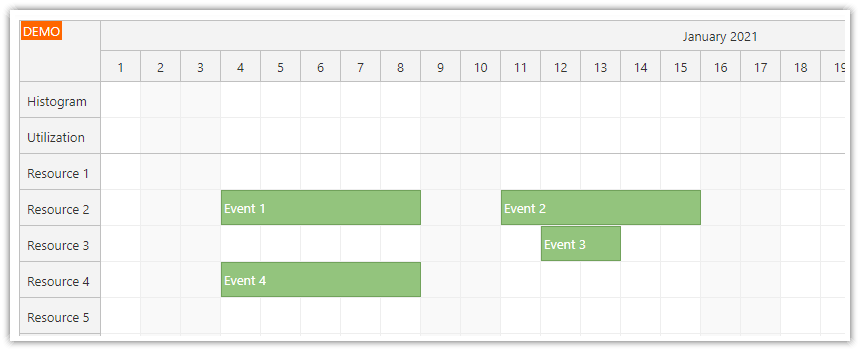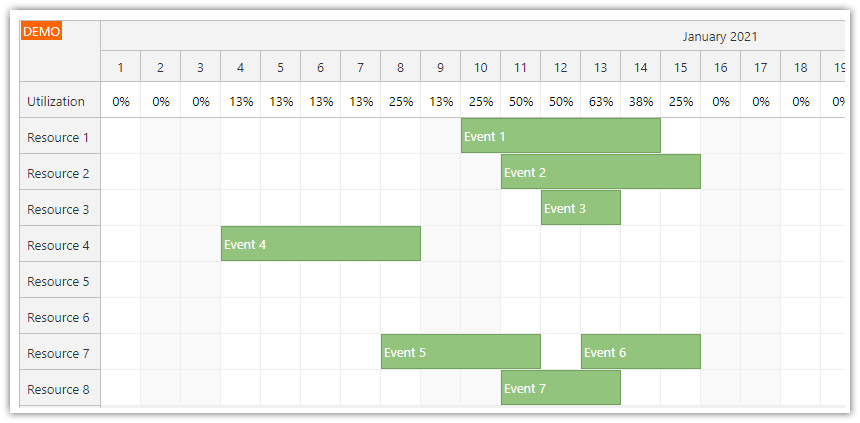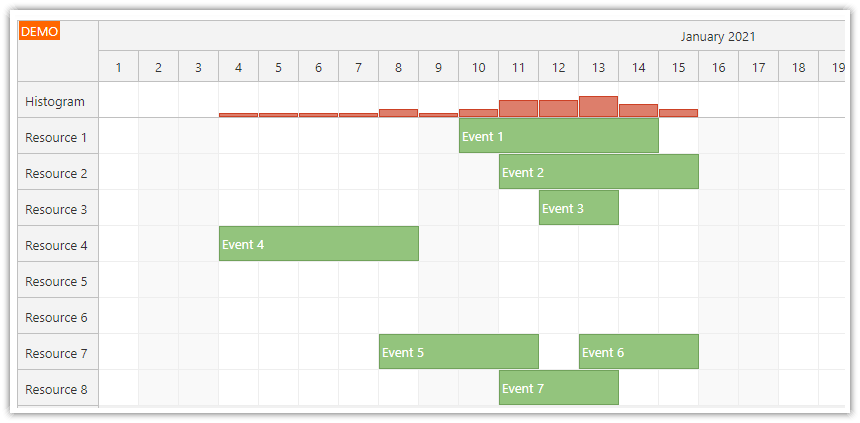## Overview

• The histogram displayed at the top of the Vue Scheduler lets you quickly evaluate the resource availability.

• Another row shows the resource utilization as percentage.

• The summary rows are fixed at the top of the Scheduler component (they don’t scroll with the content and stay always visible).

• This tutorial assumes you already know how to install and configure the Vue Scheduler component (for an introduction, please see Vue Scheduler: Build a Reservation Application in 5 Minutes).

• The Vue project includes a trial version of DayPilot Pro for JavaScript (see License below).

Licensed for testing and evaluation purposes. Please see the license agreement included in the sample project. You can use the source code of the tutorial if you are a licensed user of DayPilot Pro for JavaScript.

## How to Add Rows with Availability Details to the Vue SchedulerThe resources displayed in the Vue Scheduler rows are defined using the `resources` property of the `config` object. It’s an array of objects with row details.

In this example, we use a statically-defined `resources` array. Normally, you would load this data using an HTTP from a database. To make things simple, we just use a plain array.

Before using the row data, we extend it with two special rows that are added to the top of the list:

• The “Histogram” row will display the histogram bars (a graphical representation of the utilization).

• The “Utilization” row will display the percentage.

The histogram rows will be displayed at the top and they will be frozen and always visible (`frozen: "top"` property).

We use the three dots operator (spread syntax) to insert the original items from the `resources` array.

As soon as the `config` object is modified, Vue will detect the change and update the Scheduler component automatically.

``````loadResources() {
const resources = [
{name: "Resource 1", id: "R1"},
{name: "Resource 2", id: "R2"},
{name: "Resource 3", id: "R3"},
{name: "Resource 4", id: "R4"},
{name: "Resource 5", id: "R5"},
{name: "Resource 6", id: "R6"},
{name: "Resource 7", id: "R7"},
{name: "Resource 8", id: "R8"},
];

this.config.resources = [
{name: "Histogram", id: "histogram", frozen: "top", cellsAutoUpdated: true, cellsDisabled: true},
{name: "Utilization", id: "utilization", frozen: "top", cellsAutoUpdated: true, cellsDisabled: true},
...resources
];
}``````

## How to Calculate the Utilization PercentageThe Vue Scheduler component lets you write custom content to the grid cells. In the following example, we will use this feature to add custom text to the “Utilization” row.

You can write to the Scheduler grid cells using `onBeforeCellRender` event. You can define the event handler using the `config` object:

``````config: {
// ...
onBeforeCellRender: args => {

},
}``````

In order to display the utilization percentage in the first row, we need to calculate the total number of rows. This line reads the Scheduler rows and filters out the summary and parent rows:

``const max = this.scheduler.rows.all().filter(r => r.data.frozen !== "top" && r.children().length === 0).length;``

Now we can count events for the time column:

``const inUse = this.scheduler.events.forRange(args.cell.start, args.cell.end).length;``

And calculate the percentage:

``const percentage = inUse / max;``

Now that we have the utilization percentage we can write it to the cell:

``````const text = Math.round(100 * percentage);
args.cell.properties.text = `\${text}%`,``````

And this is our `onBeforeCellRender` implementation that calculates and displays the utilization in the first Scheduler row:

``````onBeforeCellRender: args => {
const max = this.scheduler.rows.all().filter(r => r.data.frozen !== "top" && r.children().length === 0).length;
const inUse = this.scheduler.events.forRange(args.cell.start, args.cell.end).length;
const percentage = inUse / max;

if (args.cell.resource === "utilization") {

const text = Math.round(100 * percentage);

args.cell.properties.backColor = "#ffffff";
args.cell.properties.text = `\${text}%`,
}

}``````

## How to Draw the Utilization HistogramIn order to display the availability graphically, we add another fixed row at the top of the Vue Scheduler. It will display a histogram - each time cell will display a red bar with a height set according to the utilization. The higher the bar, the higher the utilization. This will let users evaluate the availability by taking a quick look.

The histogram bars are displayed using active areas (`args.cell.properties.areas`). We only display the bar if there is any event in that time column (`inUse > 0`).

``````onBeforeCellRender: args => {
const max = this.scheduler.rows.all().filter(r => r.data.frozen !== "top" && r.children().length === 0).length;
const inUse = this.scheduler.events.forRange(args.cell.start, args.cell.end).length;
const percentage = inUse / max;

if (args.cell.resource === "histogram") {
args.cell.properties.backColor = "#ffffff";
if (inUse > 0) {

const cellHeight = this.scheduler.eventHeight - 1;
const barHeight = Math.min(percentage, 1) * cellHeight;

args.cell.properties.areas = [
{
bottom: 1,
height: barHeight,
left: 0,
right: 1,
backColor: "#dd7e6b",
style: "box-sizing: border-box; border: 1px solid #cc4125;",
}
];
}
}

}``````